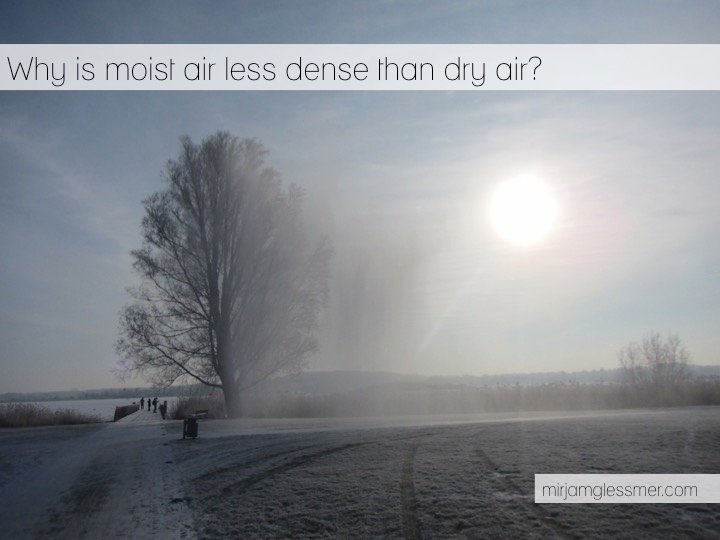# Tag Archives: air# Why moist air is lighter than dry air.

Why is moist air lighter than dry air? This seems pretty counter-intuitive at first, but then really isn’t.

I promised to do a post on why moist air is lighter than dry air a long time ago, and wrote it about a year and a half ago (!), but never published it. So here we go now!

First, we need to assume that air is an ideal gas. In that case, the number of molecules in a given volume depends only on the pressure and temperature of the gas. This is given in the ideal gas law:

PV=NkT

with P the pressure, V the volume, N Avogadro’s number = 6.0221 x 1023 /mol, k the Bolzman constant 1.38066 x 10-23 J/K and T the absolute temperature.

Is the assumption that air is an ideal gas a good one? Despite my sister’s insistence, I am not going to write a post on how I dyed all molecules in a volume of air and counted them (very funny, ha ha). So experimentally confirming N or k isn’t going to happen. But we can qualitatively show that if the number of gas molecules increases and the temperature stays the same, pressure and/or volume have to increase. We can also show that if we change the volume, this will affect pressure and temperature. All of those experiments might happen in a future post, they are all pretty standard and not very exciting.

Assuming that the ideal gas law holds for air, this means that since the number of molecules per volume is constant, the density depends on the mass of the molecules inside the volume.

Air contains a lot of N2 and O2. N’s atomic unit mass is 14, O’s is 16. N2 and O2 hence are heavy molecules with N2 weighing 28 and O2 32 atomic units. Water vapor are water molecules, and the atomic weight of H2O is 16+1+1 = 18. Each water vapor molecule is hence a lot lighter than any N2 or O2 molecule, and since the total number of molecules per volume at a given temperature and pressure is constant, the more H2O molecules replace N2 or O2 molecules, the moister – and lighter – the air gets.